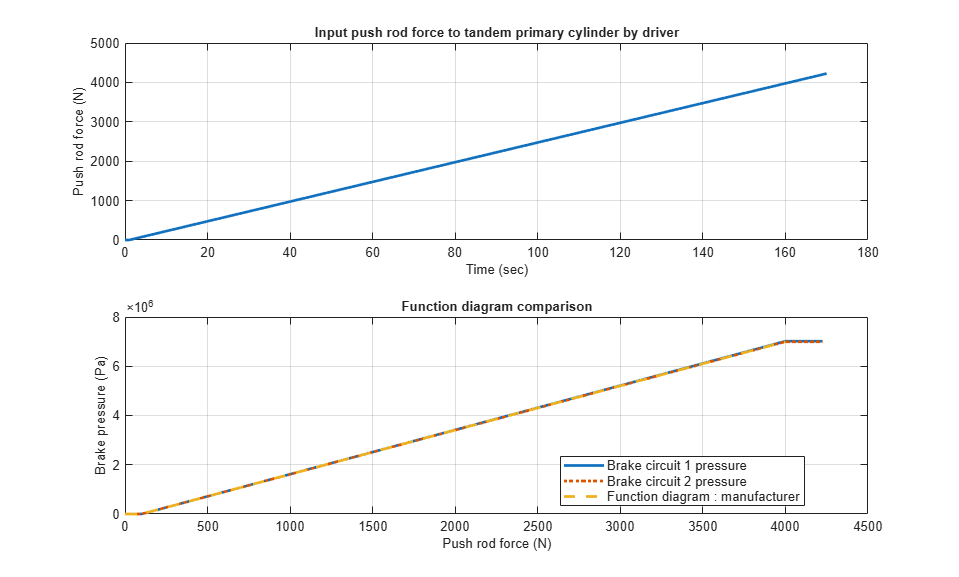# Tandem Primary Cylinder

This example shows how to model, parameterize and test a tandem primary cylinder starting from manufacturer datasheet information. First, there is a brief discussion on the mathematical modeling of the system. Given the numerical data extracted from the datasheet, optimization is then used to determine remaining unknown parameters. The model is then simulated and the resulting push rod force - brake pressure relationship curve is compared with the curve provided on the manufacturer datasheet. Understanding the behavior of the tandem primary cylinder is an important prerequisite to selection of other braking system components.

### Model

The following figure shows the tandem primary cylinder test harness. Spring-loaded accumulators are used for loading in theand thecircuits in place of disk or drum brakes. The input to the model is the push rod force coming from the lever mechanism or the brake booster. Output of the model is the pressures achieved in the brake circuits 1 and 2 respectively.### Manufacturer Datasheet Data

The supplier has provided the following data in the datasheet.

1. dp : first and second circuit piston diameter.

2. stroke1 : first circuit piston stroke.

3. stroke2 : second circuit piston stroke.

4. totalStroke : overall stroke.

5. disp1 : first circuit displacement.

6. disp2 : second circuit displacement.

7. totalDisp : net displacement.

8. maxPress : max pressure.

### Pressure Development Characteristics

The supplier datasheet gives the following function diagram or the push rod force-brake pressure curve for the tandem primary cylinder.### Equations of Motion (EoM) of System

The following figure shows the tandem primary cylinder. Mass 1 and mass 2 use the coordinate frames shown below. This description only includes the mechanical EoM of the system. The fluid dynamics equations are not shown., when, when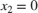, when, when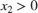where,andare positions of piston mass 1 and mass 2 from the left side assumed hard stop.andare masses of piston 1 and 2 respectively.and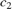are damping coefficients of piston 1 and 2 respectively.and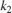are stiffness coefficients of left (Spring 1) and right (spring 2) respectively.is force applied on push rod of primary cylinder.andare pressures in brake circuit 1 and 2 respectively.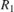and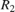are reaction forces on mass 1 and 2 respectively when both masses are at the leftmost position.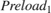andare preloads on left and right side springs respectively.

### Parameter Estimation Method

Some parameters, which are important for functionality assessment, are not provided in the manufacturer's datasheet, therefore to simulate the model it is pertinent to estimate these parameters. The EoM are converted to steady state equations to estimate unknown parameters., when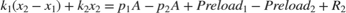, when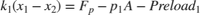, when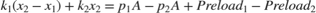, whenData for the function diagram is explained with following figure.where,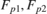andare the push rod forces for points 1,2, and 3 respectively.,Hereis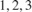for the brake circuit pressures at points 1,2, and 3 respectively.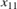andrepresent the leftmost positions for piston 1 and 2 respectively.and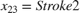as per the manufacturer datasheet data.and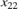are piston positions at data point 2. This point needs to be iterated on during optimisation to estimate the unknown parameters.

The estimation scheme is as follows: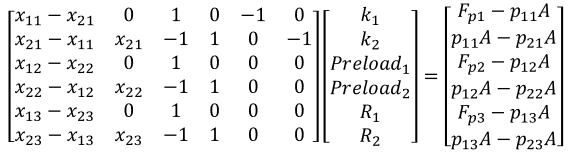The estimation should yield results with the following conditions met:

1.andshould be greater than or equal to 0.

2.andshould be greater than 0.

### Assumed Parameters

Some parameters are assumed as they are neither given in the datasheet nor can they be estimated. The following is the list:

1. c1 is damping coefficient for piston 1.

2. c2 is damping coefficient for piston 2.

3. mass1 is piston 1 mass.

4. mass2 is piston 2 mass.

5. v2D is brake circuit 1 dead volume.

6. v2M is brake circuit 1 maximum volume.

7. v4D is brake circuit 2 dead volume.

8. v4M is brake circuit 2 maximum volume.

9. a1Ori is compensating orifice area.

10. a2Ori is brake circuit orifice area

It is important to test the system with the correct load. In the test model, loading is done on the tandem primary cylinder using spring-based accumulators. Setting up correct parameters in the accumulators is important. From the datasheet it is known what should be the stroke values for brake circuits 1 and 2 respectively. Iterated parametersandare important for deciding the fluid chamber capacity of the accumulators. Assumed or estimated parameters for the load circuit accumulators are:

1. vol1 is fluid chamber capacity for brake circuit 1.

2. vol2 is fluid chamber capacity for brake circuit 2.

3. loadP1 is pressure at full capacity for brake circuit 1.

4. loadP2 is pressure at full capacity for brake circuit 2.

### Simulation Results from Simscape Logging

The model generates push rod force-brake pressure plots for selected manufacturer designs. The applied push rod force to the tandem primary cylinder is ramped at a 25 N/sec rate in the simulation.